## Fruit Drop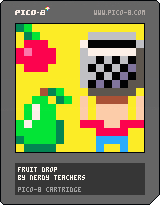Follow along with the video to make this game yourself!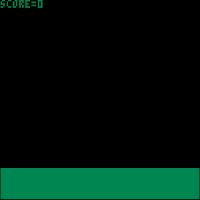The video moves quickly so pause whenever you need.

Make sure you understand why this code works or you won't be able to make your own games later!

After you made the game, change it up! Change the sprites, fiddle with the numbers, and even add to the game to see what you can create!

In making this game, we learn about drawing sprites, drawing a rectangle, using coordinates X and Y, player controls Left and Right, using a table, simple level change, and simple collision!

This lesson focuses on TABLES!

### Challenges!

1. Add a background using the map!

2. Change the fruit to something else and add more of them.

3. Set limitations to the player's movement so they stay on the screen.

4. Add something dangerous that falls and minus points if it is caught in the basket!

# Bite-size Game: Fruit Drop

## Game Sprites!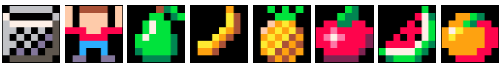What is a sprite?

You can copy these sprites or make your own! Make sure you draw each of these sprites in the exact same place, because the code will look for their sprite number. But once you understand the code, you can draw as many sprites as you want, where ever you want!

• ## 1. Set up the Variables

What is a variable?

The first code we write at the top of the file are the variables. These help us set up the game and keep track of data.

``````basket_sprite=1
player_sprite=2

player_x=64
player_y=100``````

The first two variables are named `basket_sprite` and `player_sprite`. Then the equals sign tells the game to remember it with whatever we write next. `basket_sprite` and `player_sprite` each hold a number and that number is the sprite # of the basket and player in the sprite sheet.

The next variables are named `player_x` and `player_y`. Together these tell the game where the player is on the screen. As the player moves, these numbers will change, but we can set the starting position here.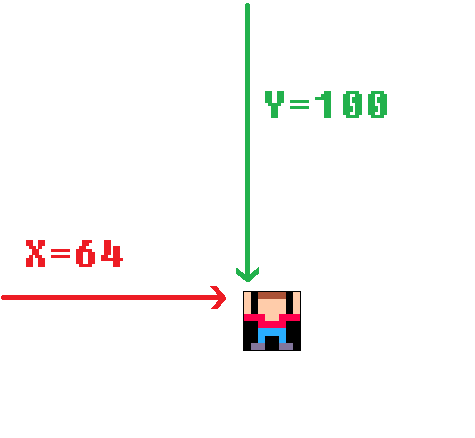`x = 64` because there are 128 pixels from left to right, so 64 is half, in the center.

`y = 100` because there are 128 pixels from top to bottom, so 100 is near the bottom.

These will be used later to start the player in the center and near the bottom of the screen.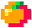The next set of variables are for keeping track of the fruit data. The best way to do this is to use a table.

``````fruits={}
fruit_start=16
fruit_count=6
fruit_interval=16``````

These `{}` are called "curly brackets" and normally look like this: { }

Curly Brackets are used to create TABLES. `fruits={}` creates an empty table. We will fill it later.

What is a Table?

`fruit_start` is the sprite number of the first fruit.

`fruit_count` is the total number of fruit sprites.

`fruit_interval` is the number of pixels between each fruit.

We will use these to create the random fruit in the game.The last set of variables are for keeping track of some game data.

``````gravity=1
level=1
points=0``````

`gravity` is the speed that the fruit will fall by.

`level` is the number of fruit that fall. Each level adds more fruit.

`points` is the player's score, how many fruit you catch.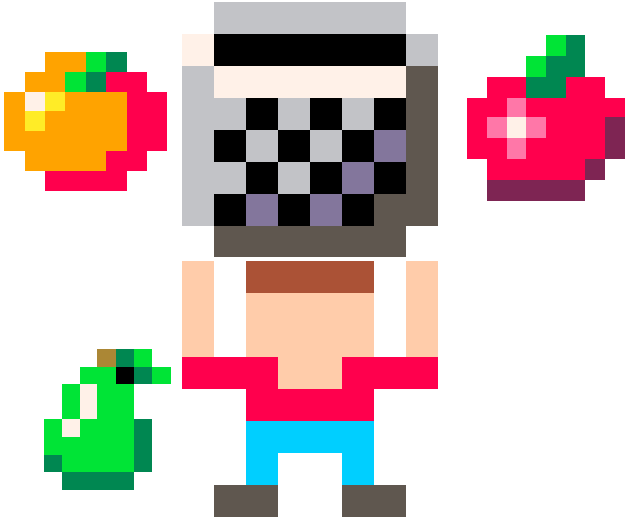• ## 2. Basic Game Functions

Next are the 3 basic functions that run the game.

``````function _init()

end

function _update()

end

function _draw()

end``````

`_init()` sets up the game.

`_update()` makes changes to the game 30 times every second.

`_draw()` runs after _update and draws to the game screen, also 30 times every second.

Basic Functions• ## 3. Draw the Player, Basket, and Ground

Let's start by drawing the player on the screen.

``````function _draw()
cls()
rectfill(0,108,127,127,3)
spr(player_sprite,player_x,player_y)
end``````

`cls()` clears the screen.

`rectfill()` draws a rectangle that is filled with a color.

`spr()` draws a sprite.

The player is drawn as `player_sprite`, which we already set as 2. The player is drawn at `player_x` and `player_y` position on the screen.

If those variables change, this draw code will change with them! That's what variables are for!The basket is drawn as `basket_sprite`, which we already set as 1. The basket is also drawn at `player_x` and `player_y` position on the screen. But we want the basket to be above the player, so we just minus 8 pixels from `player_y` because the basket is 8 pixels tall.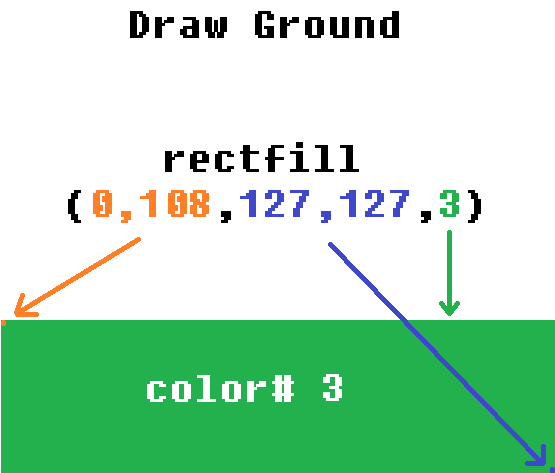The ground is created by `rectfill()` which draws a filled rectangle. The first two numbers are the rectangle's X and Y COORDINATES at the top-left corner. The next two numbers are the rectangle's X and Y COORDINATES at the bottom-right corner. The last number is the COLOR NUMBER.• ## 4. Player Controls

Next, we are updating the player controls!

``````function _update()
if btn(0) then player_x-=2 end
if btn(1) then player_x+=2 end
end``````

`btn()` (button) checks for a certain button that is pressed. `pico-8` has 6 buttons, #0-5.

`btn(0)` checks for button LEFT.

`btn(1)` checks for button RIGHT.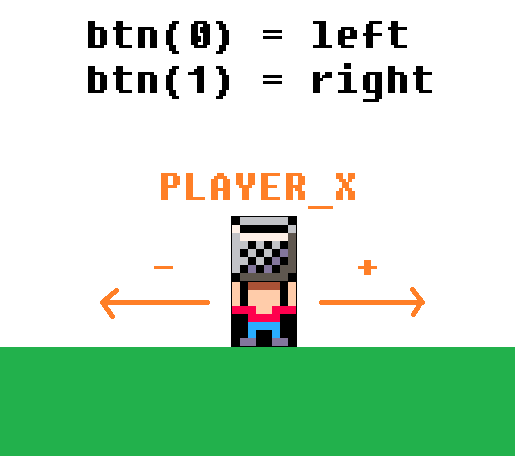To make the player sprite move based on the real player's controls, we just change the `player_x` and `player_y` variables up or down, and the sprite is drawn to match those changes.

In this game, the player only moves left and right, so only X changes and Y stays the same. If the player moves left, then the X should decrease (-) to a lower number. If the player moves right, then the X should increase (+) to a higher number.• ## 5. Create the Fruit

Math time! Don't worry, it really is simpler than it looks.

``````function _init()
for i=1,level do
fruit={
sprite=flr(rnd(fruit_count)+fruit_start),
x=flr(rnd(120)+5),
y=i*(-fruit_interval)
}
end
end``````

In the set up of the game (the `init` function), we will add fruit to the fruits table.

Each fruit will need a `SPRITE` number, an `X` coordinate, and a `Y` coordinate. Remember from when we drew the player sprite with `SPR()` that these three things are needed to draw any sprite to the screen.

First, we need to create a LOOP. This just repeats the same code a certain number of times so we don't have to copy paste code over and over again.

This is a FOR loop.

``````for i=1,level do

end``````
What is a FOR loop?

`i` is a variable that is usually used for a whole number that will count up or down. It counts the number of times this loop repeats.

`i=1` sets the variable to start at 1, and it will automatically count up by 1 each time the loop repeats.

`level` is the variable we created at the top. We will use it to keep track of the levels in the game, as the game gets harder. We will use it here to tell this FOR LOOP when to stop repeating. The way the FOR LOOP works is, it will check if `i` is more than `level`, then the loop will not repeat anymore.

This is the code inside the loop that is going to repeat. Here's how we make many fruit!

``````fruit={
sprite=  ,
x=  ,
y=
}

`fruit={...}` starts a new table to hold data unique to each fruit: SPRITE #, X, and Y.

`add(fruits,fruit)` inserts into the `fruits` table, whatever data is held in `fruit`. We will be creating a table with other tables inside it! It sometimes gets confusing but it makes coding a lot easier! You'll see!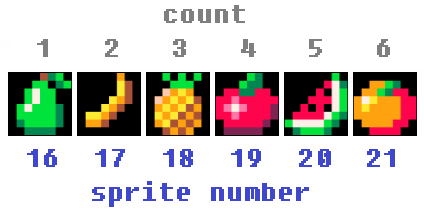For the sprite number of each fruit we do:

``flr(rnd(fruit_count)+fruit_start)``

`flr()` rounds a decimal down to the nearest whole number. Example: `flr(4.6)` rounds down to 4.

`rnd()` creates a random number between 0 and the number inside the ( ). Example: `rnd(10)` could be 0.4 or 9.345 but never more than 10.

`rnd(fruit_count)` means we want to get a random number between 0 and the total number of fruit.

But that will give us a number between 0 and 6, and we don't have fruit sprites in those low numbers. Our fruit sprites start at sprite number 16! So...

`rnd(fruit_count)+fruit_start` will take the random number between 0 and 6, then add 16 to it! Now we will get a number between 16 and 22!

Then we use `flr()` to round the random decimal number between 16 and 22 down to a whole number, so it gives one of these numbers: {16,17,18,19,20,21}, which will match the sprite number of a fruit!

For the X coordinate of each fruit we do:

``flr(rnd(120)+5)``

`rnd(120)+5` gets a random decimal number between 5 and 125.

This will position the fruit somewhere horizontally inside of the screen. And again, `flr()` will round down to make it a whole number.

For the Y coordinate of each fruit we do:

``i*(-fruit_interval)``

`i*(-fruit_interval)` gives a number that is a certain amount less than the previous number.

Remember that `i` increases with each run of the FOR LOOP. So on the first run, `i` is 1, but on the second run, `i` is 2. So let's change those variables for the numbers they represent and show the math.

On the first loop: `i=1` and `fruit_interval=16`

So `i*(-fruit_interval)` means 1 times -16 which equals -16

On the second loop: `i=2` and `fruit_interval=16`

So `i*(-fruit_interval)` now means 2 times -16 which equals -32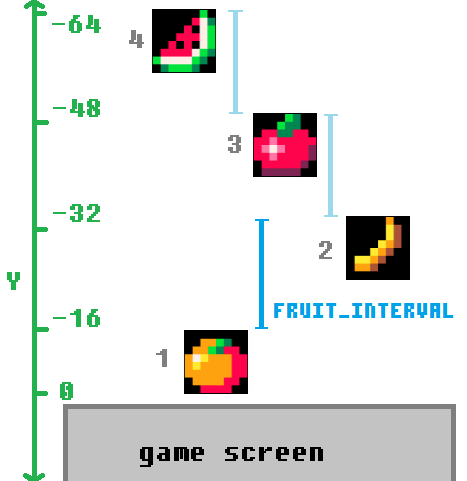So the first fruit in the table will be at Y (height) of -16 which is above the game screen. And the second fruit will be higher than that one, at -32. And the next fruit will be 16 pixels above the last one. On and on.• ## 6. Update the Fruit

This is where we update all of the fruit.

``````function _update()
for fruit in all(fruits) do

end
end``````

`for fruit in all(fruits)` is a special TABLE loop.

This will repeat over each item inside of the table we give it, which is: `fruits`. It also sets the item it is currently on as a variable we set, which we named: `fruit`.

But if you remember, each item in the `fruits` table, is also a table! Don't worry, this is where tables shine at holding lots of data.

This is what the `fruits` table looks like with each item as a table named `fruit`.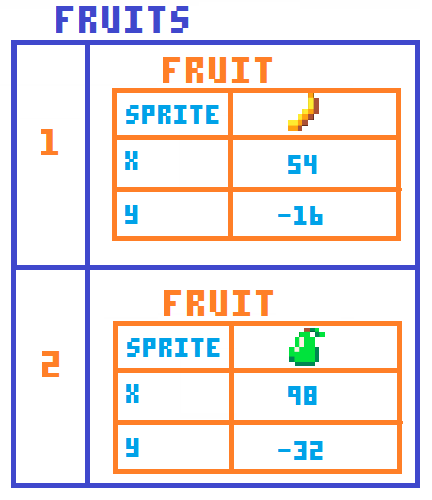The first thing we want to update about the fruit is to make them fall down the screen. So that means changing their Y, by adding to it. So inside of our table loop:

``fruit.y+=gravity``

`fruit.y` is how we access the data inside the inner table.

`+=gravity` means add to the number it already is by the number set in the variable `gravity`.

So let's take the table in the picture as an example. The first fruit is a banana, with a Y of -16. And remember the gravity that we set is 1. So `fruit.y+=gravity` just means `-16+1`. And it will update this 30 times a second because of `_update()`. So every update the banana will move 1 pixel down the screen, and after 1 second, the banana will have moved 30 pixels!

With this set up, the higher you set the `gravity`, the faster the fruit will fall! Try it out!

The next code inside of this table loop detects if the fruit fall into the basket!

``````if  fruit.y+4>=player_y-8
and fruit.y+4<=player_y
and fruit.x+4>=player_x
and fruit.x+4<=player_x+8 then
points+=1
del(fruits,fruit)
end``````

This type of code is basic "collision detection". In other words, checking if one thing hits another. In this case, we want to know if the middle of the fruit is within the limits of the basket.

`fruit.y+4` and `fruit.x+4` tell us the middle of the sprite because we know that the fruit are each 8 pixels wide and tall.

`player_x` and `player_y` help us create limits, which is a box (a hitbox!) to compare the fruit position with to know if the fruit is inside of it.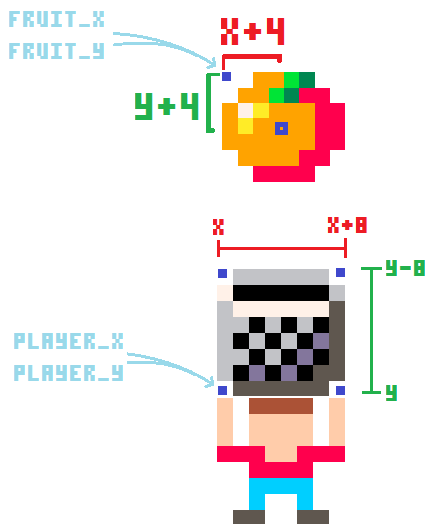If that is true, then the fruit IS inside the basket, so we increase the player's points and delete that fruit.

`points+=1` will take whatever the points is and increase it by 1.

`del()` deletes an item from a table.

`del(fruits,fruit)` deletes from the `fruits` table, the current item we are looping on: `fruit`.

The last thing inside of this table loop deletes fruit that hit the ground.

``````if fruit.y>100 then
del(fruits,fruit)
end``````

If the Y of the fruit is more than 100 (about where the ground is) then delete that fruit from the fruits table.

We are done with the table loop. But the last thing we need to update is when there are no more fruit in the game, then we increase the level and reset the fruit table.

``````if #fruits==0 then
level+=1
_init()
end``````

`#` (#) counts the items in a table.

`if #fruits==0` checks if the number of items in the fruits table is 0. In other words, if the fruits table is empty, and there are no more fruit in the game.

`level+=1` takes whatever number `level` is and increases it by 1.

`_init()` triggers the code inside the function named `_init`. That is the code that creates fruit and puts them in the fruits table. Since we just increased the level, when we run that code again, the number of fruit put in the game will also increase.• ## 7. Draw the Fruit and Score

LAST THING TO DO!!!!

``````function _draw()
--after drawing ground and sprites--

for fruit in all(fruits) do
spr(fruit.sprite,fruit.x,fruit.y)
end

print("score= "..points)
end``````

`for fruit in all(fruits) do` is the same table loop we used in `_update` to repeat over each fruit.

`spr(fruit.sprite,fruit.x,fruit.y)` draws the sprite#, X, and Y of the fruit that is currently selected by the table loop.

What is print()?

`print()` writes to the game screen.

`print("score=")` writes the exact characters inside the " ", called a STRING.

`..` will combine (CONCATENATE) two things next to each other.

`print("score="..points)` writes on the screen: the STRING "score=" then the number held by the variable `points`.

Well done! That's everything you need to know about how this game code works! Now test your understanding by playing the game and changing the code to make it your own! Have fun!• ## Full Code!

``````
player_sprite=2

player_x=64
player_y=100

fruits={}
fruit_start=16
fruit_count=6
fruit_interval=16

gravity=1
level=1
points=0

function _init()
for i=1,level do
fruit={
sprite=flr(rnd(fruit_count)+fruit_start),
x=flr(rnd(120)+5),
y=i*(-fruit_interval)
}
end
end

function _update()
if btn(0) then player_x-=2 end
if btn(1) then player_x+=2 end

for fruit in all(fruits) do
fruit.y+=gravity

if  fruit.y+4>=player_y-8
and fruit.y+4<=player_y
and fruit.x+4>=player_x
and fruit.x+4<=player_x+8 then
points+=1
del(fruits,fruit)
end

if fruit.y>100 then
del(fruits,fruit)
end
end

if #fruits==0 then
level+=1
_init()
end
end

function _draw()
cls()
rectfill(0,108,127,127,3)
spr(player_sprite,player_x,player_y)

for fruit in all(fruits) do
spr(fruit.sprite,fruit.x,fruit.y)
end

print("score= "..points)
end
``````

•• ## Play the Game!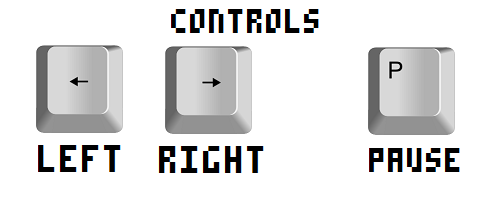`font`